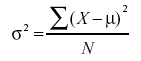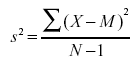# What is Variability

Variability refers to

• how much the numbers in a distribution differ from each other.
• how "spread out" a group of scores is.

The terms variability, spread, and dispersion are synonyms, and refer to how spread out a distribution is.

Quiz Example

Quiz1

Quiz2

• The graphs above represent the scores on two quizzes.
• The mean score for each quiz is 7.0.
• Despite the equality of means, you can see that the distributions are quite different.
• Specifically, the scores on Quiz 1 are more densely packed and those on Quiz 2 are more spread out.
• The differences among students were much greater on Quiz 2 than on Quiz 1.

# Measures of Variability

There are four frequently used measures of variability: the range, interquartile range, variance, and standard deviation.

## Range

• The range is the simplest measure of variability to calculate, and one you have probably encountered many times in your life.
• The range is simply the highest score minus the lowest score.

Example 1

• What is the range of the following group of numbers: 10, 2, 5, 6, 7, 3, 4?
• The highest number is 10, and the lowest number is 2, so 10 - 2 = 8.
• The range is 8.

Example 2

• Here’s a dataset with 10 numbers: 99, 45, 23, 67, 45, 91, 82, 78, 62, 51.
• The highest number is 99 and the lowest number is 23, so 99 - 23 equals 76; the range is 76.

Quiz Example

• Vonsider the two quizzes shown in the graphs above.
• On Quiz 1, the lowest score is 5 and the highest score is 9; the range is 4.
• On Quiz 2, the lowest score is 4 and the highest score is 10; the range is 6.
• The range on Quiz 2 was larger

## Interquartile Range

• The interquartile range (IQR) is the range of the middle 50% of the scores in a distribution.
• It is computed as follows:
```IQR = 75th percentile - 25th percentile
```

Quiz Example

• For Quiz 1, the 75th percentile is 8 and the 25th percentile is 6; The interquartile range is 2.
• For Quiz 2, which has greater spread, the 75th percentile is 9, the 25th percentile is 5, and the interquartile range is 4.
• In box plots, the 75th percentile was called the upper hinge and the 25th percentile was called the lower hinge.
• Using this terminology, the interquartile range is referred to as the H-spread.

### semi-interquartile range

• The semi-interquartile range is defined simply as the interquartile range divided by 2.
• If a distribution is symmetric, the median plus or minus the semi-interquartile range contains half the scores in the distribution.

## Variance

• Variability can also be defined in terms of how close the scores in the distribution are to the middle of the distribution.
• Using the mean as the measure of the middle of the distribution, the variance is defined as the average squared difference of the scores from the mean.

### Population Variance

The formula for the variance is:

```where σ2 is the variance, μ is the mean, and N is the number of numbers
```
File:ClipCapIt-140527-093540.PNG

Quiz Example

The data from Quiz 1 are shown in Table below.

Scores Deviation from Mean Squared Deviation
9 2 4
9 2 4
9 2 4
8 1 1
8 1 1
8 1 1
8 1 1
7 0 0
7 0 0
7 0 0
7 0 0
7 0 0
6 -1 1
6 -1 1
6 -1 1
6 -1 1
6 -1 1
5 -2 4
5 -2 4
Means
7 0 1.5
• The mean score is 7.0.
• Therefore, the column "Deviation from Mean" contains the score minus 7.
• The column "Squared Deviation" is simply the previous column squared.
• The mean deviation from the mean is 0. This will always be the case.
• The mean of the squared deviations is 1.5.
• Therefore, the variance is 1.5.
• Analogous calculations with Quiz 2 show that its variance is 6.7.
• Using the formula, for Quiz 1, μ = 7 and N = 20.

### Sample Variance

• If the variance in a sample is used to estimate the variance in a population, then the formula for Population Variance underestimates the variance and the following formula should be used is below.
```where s2 is the estimate of the variance and M is the sample mean.
```
• Note that M is the mean of a sample taken from a population with a mean of μ.
• Since, in practice, the variance is usually computed in a sample, this formula is most often used.

Example

• Assume the scores 1, 2, 4, and 5 were sampled from a larger population.
• To estimate the variance in the population you would compute s2 as follows:
``` M = (1 + 2 + 4 + 5)/4 = 12/4 = 3.
s2 = [(1-3)2 + (2-3)2 + (4-3)2 + (5-3)2]/(4-1)
= (4 + 1 + 1 + 4)/3 = 10/3 = 3.333
```

### Alternate Formulas

• There are alternate formulas that can be easier to use if you are doing your calculations with a hand calculator.
• These formulas are subject to rounding error if your values are very large and/or you have an extremely large number of observations.

For the example above,

## Standard Deviation

• The standard deviation is the square root of the variance.

Quiz Example The standard deviations of the two quiz distributions 1.225 and 2.588.

### Standard Deviation and Normal Distribution

• The standard deviation is an especially useful measure of variability when the distribution is normal or approximately normal because the proportion of the distribution within a given number of standard deviations from the mean can be calculated.
• 68% of the distribution is within one standard deviation of the mean and approximately 95% of the distribution is within two standard deviations of the mean.
• Therefore, if you had a normal distribution with a mean of 50 and a standard deviation of 10, then 68% of the distribution would be between 50 - 10 = 40 and 50 +10 =60.
• Similarly, about 95% of the distribution would be between 50 - 2 x 10 = 30 and 50 + 2 x 10 = 70.
• The symbol for the population standard deviation is σ; the symbol for an estimate computed in a sample is s.

Example

Figure above shows two normal distributions.

• The red distribution has a mean of 40 and a standard deviation of 5
• The blue distribution has a mean of 60 and a standard deviation of 10
• For the red distribution, 68% of the distribution is between 35 and 45
• for the blue distribution, 68% is between 50 and 70.

## Quiz

<quiz display=simple > { What is the range of 2, 4, 6, and 8?

|type="{}"} { 6 }

{

6

8 - 2 is 6

}

{Would the variance of 10, 12, 17, 20, 25, 27, 42, and 45 be larger if the numbers represented a population or a sample?

|type="()"} -Population +Sample

{

Sample

The variance would be larger if these numbers represented a sample because you would divide by N-1 (instead of just N).

}

{What is the standard deviation of this sample?

```Y
8
15
20
12
13
11
13
15
```

|type="{}"} { 3.5026 }

{

3.5026

}

{What is the interquartile range of these numbers?

```Z
12
13
14
15
9
10
16
10
8
10
11
12
13
22
23
24
25
```

|type="{}"} { 9 }

{